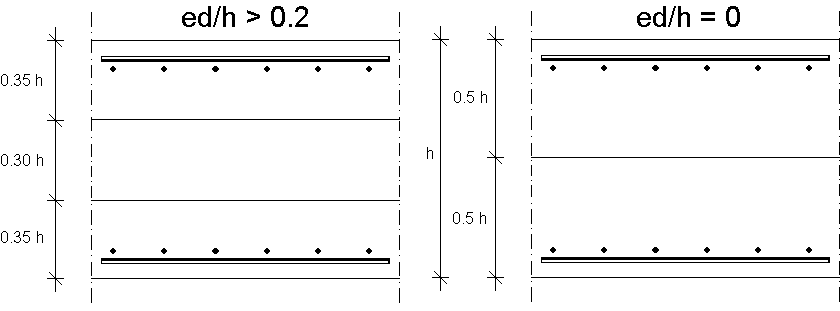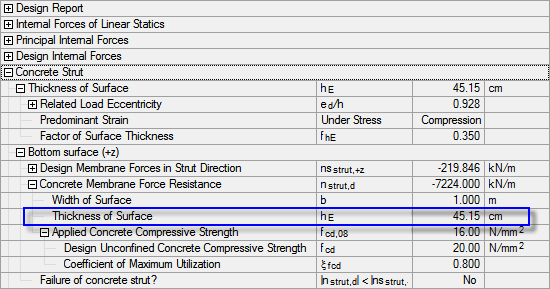# RF-CONCRETE Surfaces – Online Manual Version 5

Online manuals, introductory examples, tutorials, and other documentation.

# 2.5.4 Analysis of the Concrete Compression Struts

### Analysis of the Concrete Compression Struts

To design the concrete compression strut of a shell, it is divided into three surface layers that are subjected to the design membrane forces.Figure 2.71 Surface layer thicknesses for shells mainly subjected to moment (left) or compression force (right)

For shells where the applied moment is relatively large in relation to the acting axial force (ed/h > 0.2), the thickness hE of the two outer layers is reduced to 0.35 ⋅ d. For shells subjected to approximately concentric compression, the surface layer thickness hE is increased to half of the plate thickness h. If the relative eccentricity of the axial force ed/h is between 0 and 0.2, the surface layer thickness is interpolated.

For ed, the larger value from the quotients of mx/nx and my/ny is applied.

For the analysis of the concrete compression strut, the concrete strut's compression force to be resisted nstrut,+z is compared with the resistant axial force of the surface layer nstrut,d.Figure 2.72 Concrete strut and thickness of surface layer

The resistant axial force nstrut,d depends on the thickness hE of the surface layer and the applied concrete strength fcd,08.

The first step to determine the thickness of the surface layer is to determine the provided load eccentricities in x- and y-direction from the internal forces of the linear plate analysis:

The greater load eccentricity in x-direction is computed as governing. It can be used to determine the relative load eccentricity ed/h.

Since the relative load eccentricity is greater than 0.2, it is a shell that is predominantly subjected to bending. The factor fhE for determining the surface layer thickness is 0.35.

Thus, the thickness hE of the surface layer is determined as follows:

The design value of the concrete compressive strength is reduced to 80 % according to the recommendations of Schlaich/Schäfer (in , page 378). This recommendation can also be found in EN 1992-1-1, clause 6.5.2, which regulates the design of compression struts in framework models.

This value can also be found in the design details (see Figure 2.72).

With it, you can determine the resisting force of the concrete compression strut nstrut,d.

• nstrut,d = b ∙ hE ∙ fcd,08 = 100 ∙ 45.15 ∙ 16 = 7 224.00 kN/m

The analysis of the concrete compression strut for the top side of the surface is done analogously.

Literatur
  Jörg Schlaich and Kurt Schäfer. Konstruieren im Stahlbetonbau. Betonkalender, 1993.